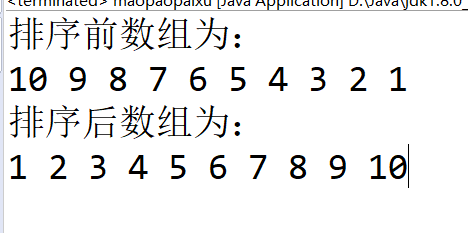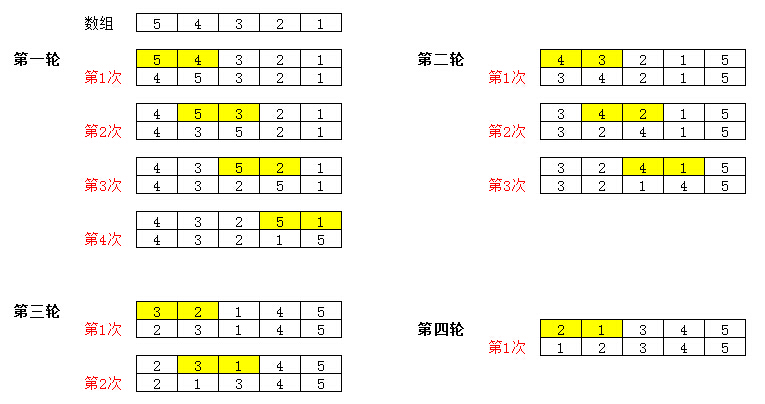• ## Java冒泡排序算法

千次阅读 多人点赞 2018-10-21 07:25:16
java冒泡排序算法 1.基本思想： 对比相邻的元素值，如果满足条件就交换元素值，把较小的元素移动到数组的前面（从小到大排序），把大的元素移动到数组的后面，即交换两个元素的位置，这样较小的元素就像气泡一样从...
java冒泡排序算法
1.基本思想：
对比相邻的元素值，如果满足条件就交换元素值，把较小的元素移动到数组的前面（从小到大排序），把大的元素移动到数组的后面，即交换两个元素的位置，这样较小的元素就像气泡一样从底部上升到顶部。
2.算法实现：
冒泡算法由双层循环实现，其中外层循环用于控制排序轮数，一般为要排序的数组长度减1，因为最后一次循环只剩下一个数组元素，不需要对比，同时已经完成排序了。内层循环主要是用于对比数组中每个邻近元素的大小，以确定是否交换位置，对比和交换的次数随排序轮数而减少。
3.代码
public class maopaopaixu {

public static void main(String[] args) {
// TODO Auto-generated method stub
Scanner input=new Scanner(System.in);
int[] array= {10,9,8,7,6,5,4,3,2,1};
System.out.println("排序前数组为：");
for(int i=0;i<array.length;i++)
System.out.print(array[i]+" ");
System.out.println();
BubbleSort(array);
System.out.println("排序后数组为：");
for(int i=0;i<array.length;i++)
System.out.print(array[i]+" ");
}

public static void BubbleSort(int[] array) {
for(int i=1;i<array.length;i++) {
for(int j=0;j<array.length-i;j++) {
int temp;
if(array[j]>array[j+1]) {
temp=array[j];
array[j]=array[j+1];
array[j+1]=temp;
}
}
}
}

}

4.运行结果展开全文• java冒泡排序算法,sort方法，main方法
• 基于java实现冒泡排序算法 算法描述： 比较相邻的两个元素，如果前面的比后面的大，则交换两个元素； 对每每相邻的元素都进行这样的比较操作，从开始的一对到最后一对，这样最后的元素会是本次遍历完剩下的最大的...
基于java实现冒泡排序算法
算法描述：

比较相邻的两个元素，如果前面的比后面的大，则交换两个元素；
对每每相邻的元素都进行这样的比较操作，从开始的一对到最后一对，这样最后的元素会是本次遍历完剩下的最大的元素；
针对所有的元素执行以上步骤，除了已经指派出来的最大的元素（或序列，序列排在了最末尾）
重复以上步骤直至排序完成。

冒泡算法进行升序排列：
public static void main(String[] args) {
// 使用冒泡法进行升序排列
int [] number = {1,3,4,2,5,9,6,8,7};
for (int i = 0; i <number.length ; i++) {
for (int j = i+1; j <number.length ; j++) {
if (number[i]>number[j]){
int change1 = number[j];
number[j] = number[i];
number[i] = change1;         //交换双方的值
}
}
}
for (int i : number) {
System.out.print(i);
}
}

冒泡算法进行降序排列
public static void main(String[] args) {
// 使用冒泡法进行升序排列
int [] number = {1,3,4,2,5,9,6,8,7};
for (int i = 0; i <number.length ; i++) {
for (int j = i+1; j <number.length ; j++) {
if (number[i]<number[j]){
int change1 = number[j];
number[j] = number[i];
number[i] = change1;         //交换双方的值
}
}
}
for (int i : number) {
System.out.print(i);
}
}


使用java中的sort方法进行进行升序降序

public static void main(String[] args) {
// 使用sort方法进行升序
int [] number3 = {1,3,4,2,5,9,6,8,7};
Arrays.sort(number3);
for (int i : number3) {
System.out.print(i);
}
System.out.println();

// 进行降序
for (int i = number3.length-1; i >=0 ; i--) {
System.out.print(number3[i]);
}
}



展开全文算法 快速排序
• 介绍了java冒泡排序算法代码，有需要的朋友可以参考一下
• 冒泡算法原理
冒泡算法原理
1.比较两个相邻的元素，如果一个大于第二个，则交换他们；否则不用交换；
2.从第一个元素开始，依次比较两个相邻的元素，直到最后一个元素；
3.重复步骤2，开始新一轮的比较，直到得出排序后的结果。Java代码如下：
package cn.com.chiclewu;

/**
* 练习冒泡排序法
* @author chiclewu
*
*/
public class BubbleSort {
//按照升序进行排序
public static void ascendingSort(int[] array) {
int temp;
for (int i = 0; i < array.length; i++) {
for (int j = 0; j < array.length - 1 - i; j++) {
if (array[j] > array[j + 1]) {
temp = array[j];
array[j] = array[j + 1];
array[j + 1] = temp;
}
}
}
}

//按照降序进行排序
public static void descendingSort(int[] array) {
int temp;
for (int i = 0; i < array.length; i++) {
for (int j = 0; j < array.length - 1 - i; j++) {
if (array[j] < array[j + 1]) {
temp = array[j];
array[j] = array[j + 1];
array[j + 1] = temp;
}
}
}
}

public static void main(String[] args) {
int[] a = { 5, 7, 2, 8, 3 };
ascendingSort(a);
System.out.println("升序：");
for (int i = 0; i < a.length; i++) {
System.out.print(a[i] + " ");
}
System.out.println();

int[] b = { 5, 7, 2, 8, 3 };
descendingSort(b);
System.out.println("降序：");
for (int i = 0; i < b.length; i++) {
System.out.print(b[i] + " ");
}

}
}

输出结果：
升序：
2 3 5 7 8
降序：
8 7 5 3 2


展开全文• 简单的总结一下冒泡排序的实现: ... * @description java冒泡排序算法 * 原理:比较两个相邻的元素,将值大的元素交换至右端 */ public class BubblingSort { public static void main(String[] args) { ...
简单的总结一下冒泡排序的实现:

package arithmetic;

/**
* @author JasonLee
* @description java的冒泡排序算法
* 原理:比较两个相邻的元素,将值大的元素交换至右端
*/
public class BubblingSort {
public static void main(String[] args) {
int arr[] = {5, 3, 1, 7, 9, 6, 2, 8};
// 排序的次数
for (int i=0;i<arr.length-1;i++){
// 每一次需要排多少次
for (int j=0;j<arr.length-1-i;j++){
// 如果第一个大于第二个,则互换两个的位置
if(arr[j]>arr[j+1]){
int temp = arr[j+1];
arr[j+1] = arr[j];
arr[j] = temp;
}
}
}
for (int num: arr){
System.out.println(num);
}
}
}



展开全文• 冒泡排序（Bubble Sort）是一种简单的排序算法。它重复地遍历要排序的数列...1.冒泡排序算法的运作如下: 1. 对每一对相邻元素作同样的工作，从开始第一对到结尾的最后一对。在这一点，最后的元素应该会是最大的数 2算法
• 冒泡排序是一种简单的排序算法。它重复地走访过要排序的数列，一次比较两个元素，如果他们的顺序错误就把他们交换过来。走访数列的工作是重复地进行直到没有再需要交换，也就是说该数列已经排序完成。这个算法的...
• 1.首先我们先测试以下，随机数目为10个，看看冒泡排序所用时间。 public class BubbleSort { /** * 创建日期：2017-3-24下午7:55:39 作者：lvguanghui */ public static void main(String[] args) { /** ...java算法 java冒泡排序 冒泡排序算法
•  System.out.println("第几次排序"+ i);    }    return arr;  }  public static void printArray(int[] arr) {  for(Integer item : arr) {  System.out.print(item+" ");  }    }    ...
• JAVA实现冒泡排序算法 》中，我们用常规算法实现了冒泡排序，在此篇中，我们对冒泡排序算法进行优化，思想如下：引入一个标志位，默认为true，如果本次或者本趟遍历前后数据比较发生了交换，则标志位设置为true，...
• 冒泡排序算法是由左向右，按顺序对两个数据比大小（如果是想数据由小到大排列），前一个比后一个大，那么就交换一下位置。每循环一轮，都会将该轮循环所遇到的最大的数排到最后面。假设数组长度是length,那么只要...算法 数据结构
• public class BubleSort { public static void print(int [] temp) {//打印数组算法 for(int i=0;i;i++) { System.out.print(" " + temp[i]); } } public static void bubleSort(intstring class
• /** * * 冒泡排序算法~~~ */public class test { public static void main(String args[]){ int arrayA[]={1,5,4,23,8,6,7,9,16,32}; int[] arrayB=sort(arrayA); for(int i=0;istring class
• 本文出自 “唐大老师” 博客，请务必保留此出处http://tscjsj.blog.51cto.com/412451/84561 public class Bubble {// 冒泡排序函数1public static void bubbleSort1(Comparable []data){ int position,scan;...string class c
• 1、什么是冒泡排序算法 冒泡排序算法是一种比较简单的排序算法，上学时候的老是都应该讲过该经典算法。 它重复地走访过要排序的元素列，依次比较两个相邻的元素，如果顺序（如从大到小、首字母从Z到A）错误就把他们...算法 数据结构 快速排序...

# java冒泡排序算法java 订阅